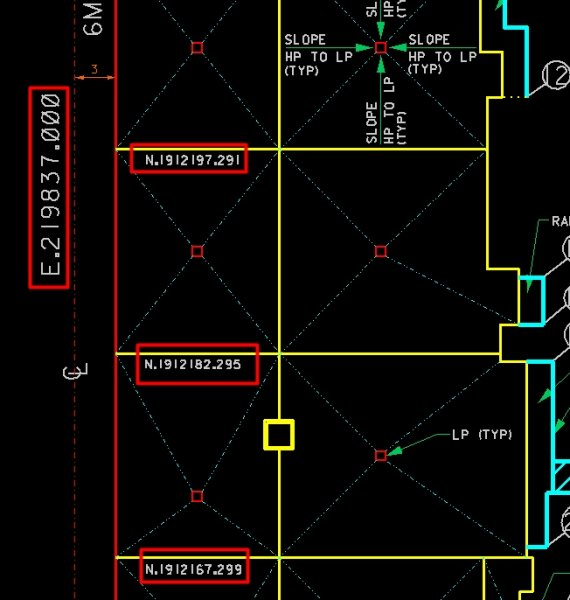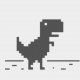# labelling horizontal line or vertical line coordinate parallel to line

## Recommended PostsDear all,

Do you have a lisp to labelling horizontal line or vertical line coordinate, just the line's Northing or Easting coordinate parallel to line ,it can be above ,under or on line, can chose location. and can labelling several lines one time.

thanks a lot.##### Share on other sitesI moved your thread to the AutoLISP, Visual LISP & DCL Forum.

##### Share on other sitesthanks SLW210

##### Share on other sitesthanks SLW210

Tips:

command: _dimordinate

also can prefix "N=" or "E=" , but it only shows positive value

Updated v1.1: works with LWPOLYLINE & POLYLINE

10.02.2015

```http://www.cadtutor.net/forum/showthread.php?90807-labelling-horizontal-line-or-vertical-line-coordinate-parallel-to-line&p=621946#post621946

;; Make Angle Readable      ;credit to ymg
(setq a (rem (+ a pi pi) (+ pi pi)))
(rem (if (< (* pi 0.5) a (* pi 1.5))
(+ a pi)
a
) ;_ end of if
(+ pi pi)
) ;_ end of rem
) ;_ end of defun

[color="red"];v1.1: works with LWPOLYLINE & POLYLINE[/color]
(defun c:test (/ _text ss 2p e lst split glc l)
;hanhphuc 09.02.2015
;label Vertical & Horizontal Grid (WCS)
(defun _text (tx lst / txsize rot pt)
(setq txsize (getvar 'textsize)
pt	 (polar	(apply 'mapcar (cons ''((a b) (* 0.5 (+ a b))) lst))
(+ rot (/ pi 2.))
(* txsize 3.0)
) ;_ end of polar
) ;_  end of setq
(entmake (list '(0 . "TEXT")
'(72 . 1) ; justify
(cons 1 tx)
(cons 10 pt)
(cons 11 pt) ; justify
(cons 40 txsize)
(cons 50 rot)
) ; list
) ;_ end of entmake
) ;_ end of defun

(defun split (lst len / l ls)
(while lst
(repeat len (setq l (cons (car lst) l) lst (cdr lst)))
(setq ls (cons (reverse l ) ls) l nil)
)
(vl-remove-if ''((x)(vl-some 'not x)) (reverse ls))
)

(defun glc (e / obj l cor) ; get *lines coordinates
;hanhphuc 2014
(setq obj (vlax-ename->vla-object e))
(foreach p '("Coordinates" "EndPoint" "StartPoint")
(if	(vlax-property-available-p obj p)
(setq l (cons p l))
) ;_ end of if
) ;_ end of foreach
(setq cor (mapcar ''((p) (vlax-get obj p)) l))
(eval	(cons 'cond
(reverse (vl-list* '(t cor)
(mapcar ''((a b)
(list
(list '= (cdr (assoc 0 (entget e))) a)
(cons 'quote (list (split (car cor) b)))
)
)
'("LWPOLYLINE" "POLYLINE" )
'(2 3)
) ;_ end of mapcar
) ;_ end of vl-list*
) ;_ end of reverse
) ;_ end of cons
) ;_ end of eval
) ;_ end of defun

(prompt "\nSelect grid lines.. ")
(setq ss (ssget ":L" '((0 . "*LINE"))))
(repeat (sslength ss)
(setq lst (glc (setq e (ssname ss 0))))
(foreach 2p	(mapcar ''((a b) (list a b)) lst (cdr lst))
(eval
(cons 'cond
(mapcar '(lambda (a b)
(list
(equal (car (setq l (mapcar a 2p))) (cadr l) 1.0e-
(cons '_text (list (strcat b (rtos (car l) 2 3)) '2p))
) ;_ end of list
) ;_ end of lambda
'("E= " "N= ") ; or '("X= " "Y= ")
) ;_ end of mapcar
) ;_ end of cons
) ;_ end of eval
(princ)
) ;_ end of foreach
(ssdel e ss)
) ;_ end of repeat
(princ)
) ;_ end of defun
```

HTH

Edited by hanhphuc
now user can label on polyline as requested

##### Share on other sitesthanks a lot!!!!

@hanhphuc ，I have test the lisp,it work very nice for "line",but it can not labelling the "ployline""3dpoly",could you please add the function to it, thanks a bunch....

##### Share on other sitesthanks a lot!!!!

@hanhphuc ，I have test the lisp,it work very nice for "line",but it can not labelling the "ployline""3dpoly",could you please add the function to it, thanks a bunch....

you are welcome xinmh

updated post#4

##### Share on other sitesyou are welcome xinmh

updated post#4

perfect!!

thanks a lot!!!

you are so warmheart!!!

##### Share on other sitesOn 2/9/2015 at 1:18 PM, hanhphuc said:

Tips:

command: _dimordinate

also can prefix "N=" or "E=" , but it only shows positive value

Updated v1.1: works with LWPOLYLINE & POLYLINE

10.02.2015

```

;; Make Angle Readable      ;credit to ymg
(setq a (rem (+ a pi pi) (+ pi pi)))
(rem (if (< (* pi 0.5) a (* pi 1.5))
(+ a pi)
a
) ;_ end of if
(+ pi pi)
) ;_ end of rem
) ;_ end of defun

[color="red"];v1.1: works with LWPOLYLINE & POLYLINE[/color]
(defun c:test (/ _text ss 2p e lst split glc l)
;hanhphuc 09.02.2015
;label Vertical & Horizontal Grid (WCS)
(defun _text (tx lst / txsize rot pt)
(setq txsize (getvar 'textsize)
pt	 (polar	(apply 'mapcar (cons ''((a b) (* 0.5 (+ a b))) lst))
(+ rot (/ pi 2.))
(* txsize 3.0)
) ;_ end of polar
) ;_  end of setq
(entmake (list '(0 . "TEXT")
'(72 . 1) ; justify
(cons 1 tx)
(cons 10 pt)
(cons 11 pt) ; justify
(cons 40 txsize)
(cons 50 rot)
) ; list
) ;_ end of entmake
) ;_ end of defun

(defun split (lst len / l ls)
(while lst
(repeat len (setq l (cons (car lst) l) lst (cdr lst)))
(setq ls (cons (reverse l ) ls) l nil)
)
(vl-remove-if ''((x)(vl-some 'not x)) (reverse ls))
)

(defun glc (e / obj l cor) ; get *lines coordinates
;hanhphuc 2014
(setq obj (vlax-ename->vla-object e))
(foreach p '("Coordinates" "EndPoint" "StartPoint")
(if	(vlax-property-available-p obj p)
(setq l (cons p l))
) ;_ end of if
) ;_ end of foreach
(setq cor (mapcar ''((p) (vlax-get obj p)) l))
(eval	(cons 'cond
(reverse (vl-list* '(t cor)
(mapcar ''((a b)
(list
(list '= (cdr (assoc 0 (entget e))) a)
(cons 'quote (list (split (car cor) b)))
)
)
'("LWPOLYLINE" "POLYLINE" )
'(2 3)
) ;_ end of mapcar
) ;_ end of vl-list*
) ;_ end of reverse
) ;_ end of cons
) ;_ end of eval
) ;_ end of defun

(prompt "\nSelect grid lines.. ")
(setq ss (ssget ":L" '((0 . "*LINE"))))
(repeat (sslength ss)
(setq lst (glc (setq e (ssname ss 0))))
(foreach 2p	(mapcar ''((a b) (list a b)) lst (cdr lst))
(eval
(cons 'cond
(mapcar '(lambda (a b)
(list
(equal (car (setq l (mapcar a 2p))) (cadr l) 1.0e-
(cons '_text (list (strcat b (rtos (car l) 2 3)) '2p))
) ;_ end of list
) ;_ end of lambda
'("E= " "N= ") ; or '("X= " "Y= ")
) ;_ end of mapcar
) ;_ end of cons
) ;_ end of eval
(princ)
) ;_ end of foreach
(ssdel e ss)
) ;_ end of repeat
(princ)
) ;_ end of defun
```

HTH

##### Share on other sitesFind this line

`(equal (car (setq l (mapcar a 2p))) (cadr l) 1.0e-`

it is missing the final integer and the closing brace

The brace is easy but the final integer (8) is a guess as this was an error that occured when the system software was upgraded. See reason for edit

`(equal (car (setq l (mapcar a 2p))) (cadr l) 1.0e-8)`

Edited by dlanorh
Reminded by LeeMac of system upgrade problem
•1

##### Share on other sitesOn 10/3/2019 at 4:43 AM, dlanorh said:

Find this line

```
(equal (car (setq l (mapcar a 2p))) (cadr l) 1.0e-```

it is missing the final integer and the closing brace

Thanks dlanorh,

it was mentioned which 8 ) became smiley was automatic removed in the code tags by the system.

p/s quite long time inactiveEdited by hanhphuc

## Join the conversation

You can post now and register later. If you have an account, sign in now to post with your account.
Note: Your post will require moderator approval before it will be visible.×   Pasted as rich text.   Paste as plain text instead

Only 75 emoji are allowed.